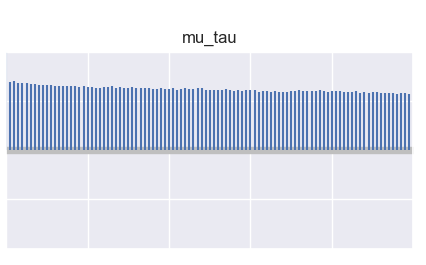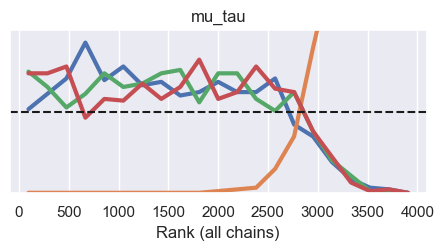# One outlier chain for hyperparameter on some random seeds

I realize that it may be hard to do much troubleshooting without the full model code, but here’s the seemingly relevant info I can easily give:

I have a hyperparameter `mu_tau` for `mu` defined roughly like this:

``````    with reparam(config={"mu_tau": TransformReparam()}):
mu_tau = (
ny.sample(
f"mu_tau",
dist.TransformedDistribution(dist.HalfNormal(scale=1), AffineTransform(loc=0, scale=0.1)),
)
)

with reparam(config={"mu": TransformReparam()}):
mu = ny.sample(
"mu",
dist.TransformedDistribution(
dist.Normal(loc=0, scale=1).expand((shape.n_endog,)),
LowerCholeskyAffine(loc=jnp.zeros(shape.n_endog), scale_tril=mu_chol * mu_tau),
),
)
``````

This model works fine (0 divergences, r hats at 1) around 70% of the time. But for some `PRNGKey`s passed to `mcmc.run`, one chain is an outlier from the rest (also happens to ~2 chains when I bump up to 8 chains). Here are the diagnostic graphs.

Latent posterior distributions for each chain:

Prior and posterior distributions:

Chain autocorrelation:Rank plot:Trace plot:

Does anyone have any guesses as to what’s going on or what additional diagnostic information I should seek?

When the chains are not mixing well, I think we need to design a better model to avoid such issue (maybe caused by multimodal posterior…). As a workaround, you can also change init strategies to avoid bad initialization.

Forgot to follow up on this:

My initial confusion here was that the issue occurred so intermittently—in the past, r hats were fairly consistent across runs.

I ended up figuring out that the problem was (I think) “multiplicative degeneracy” where observed values at or near 0 could correspond to a 0 in the parameter or a 0 in the hyperparameter. I fixed this by adding a small constant to the hyperparameter value.

1 Like